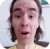FreeKB - Perl Hash (determine if hash is defined)
Perl - Hash (determine if hash is defined)Let's say you create an empty hash, like this.

``my %hash = ();``

Dumper can be used to display the structure of the hash.

``````use Data::Dumper;
print Dumper \%hash;``````

Which will display the data structure of the hash.

``\$VAR1 = {};``

The following if statement will return "%hash is not defined".

``````if (%hash) {
print "\%hash is defined\n";
}
else {
print "\%hash is not defined\n";
}``````

Let's create a key.

``\$hash{name_key} = "";``

Dumper will return the following.

``````\$VAR1 = {
'name_key' => ''
};``````

The following if statement will now return "%hash is defined". This shows that a hash becomes defined once the hash contains a key.

``````if (%hash) {
print "\%hash is defined\n";
}``````

However, the following if statement will return "\$hash{'name_key'} contains no value".

``````if (\$hash{"name"}) {
print "\\$hash{'name_key'} contains a value\n";
}
else {
print "\\$hash{'name_key'} contains no value\n";
}``````

Let's append a value to name_key.

``\$hash{name_key} = "John Doe";``

Dumper will return the following.

``````\$VAR1 = {
'name_key' => 'John Doe'
};``````

The following if statement will return "%hash is defined".

``````if (%hash) {
print "\%hash is defined\n";
}``````

The following if statement will return "\$hash{'name_key'} contains a value".

``````if (\$hash{"name"}) {
print "\\$hash{'name_key'} contains a value\n";
}``````

We will never share your name or email with anyone. Enter your email if you would like to be notified when we respond to your comment.

Please enter 8cd0b in the box below so that we can be sure you are a human.

Web design by yours truely - me, myself, and I   |   jeremy.canfield@freekb.net   |